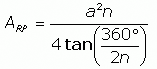SEARCH HOMEMath Central Quandaries & QueriesQuestion from Nancy: I have to submit my approximate sq. meters of an Octagon home in Costa Rica. All I know is all sides are equal. One structure has all sides at 16 feet and the second one has all sides equal at 8 ft. I then have to convert it to sq. meters. Can anybody help me with this? Thank you so much, NancyHi Nancy,

If you mean a regular octagon then I can help. for a regular octagon all eight sides must be the same length and all the angles are equal.

Look at the response that Stephen gave to Dana for her house with 14 side. Stephen developed an expression for the area ARP of a regular polygon with n sides of length a units. His expression isFor your situation n = 8 and tan(360o/16) = tan(22.5o) = 0.41421.

Since you have the side lengths in feet this will give you an area in square feet. Google can perform the conversion. If your calculations give an area of A square feet then type A square feet in square meters into the Google search window and Google will perform the conversion.

I hope this helps,
PennyMath Central is supported by the University of Regina and The Pacific Institute for the Mathematical Sciences.